# Power Factor Improvement

## Power Factor Improvement:

The low power factor is mainly due to the fact that most of the power loads are inductive and, therefore, take lagging currents. In order for Power Factor Improvement, some device taking leading power should be connected in parallel with the load. One of such devices can be a capacitor. The capacitor draws a leading current and partly or completely neutralizes the lagging reactive component of load current. This raises the power factor of the load.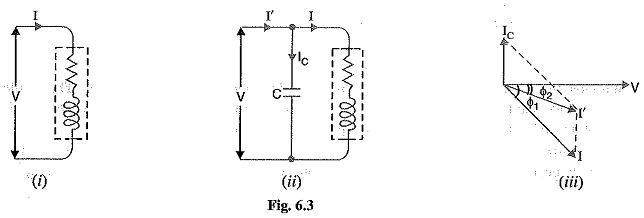Illustration: To illustrate the power factor improvement by a capacitor, consider a single phase load taking lagging current I at a power factor cos Φ1 as shown in Fig. 6.3.

The capacitor C is connected in parallel with the load. The capacitor draws current Ic which leads the supply voltage by 90°. The resulting line current I′ is the phasor sum of I and IC and its angle of lag is Φ2 as shown in the phasor diagram of Fig. 6.3. (iii). It is clear that Φ2 is less than Φ1, so that cos Φ2 is greater than cos Φ1. Hence, the power factor of the load is improved. The following points are worth noting :

1.The circuit current I′ after p.f. correction is less than the original circuit current I.

2.The active or wattful component remains the same before and after p.f. correction because only the lagging reactive component is reduced by the capacitor.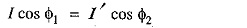3.The lagging reactive component is reduced after pl. improvement and is equal to the differ­ence between lagging reactive component of load (I sin Φ1) and capacitor current (IC) i.e.,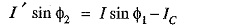Therefore, active power (kW) remains unchanged due to power factor improvement.

5.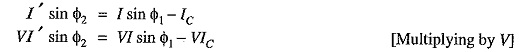i.e., Net kVAR after p.f. correction = Lagging kVAR before p.f. correction — leading kVAR of equipment

### Power Factor Improvement Equipment

Normally, the power factor of the whole load on a large generating station is in the region of 0.8 to 0-9. However, sometimes it is lower and in such cases it is generally desirable to take special steps to improve the power factor. This can be achieved by the following equipment :

1. Static capacitors.
2. Synchronous condenser.

1.Static capacitor: The power factor can be improved by connecting capacitors in parallel with the equipment operating at lagging power factor. The capacitor (generally known as static capacitor) draws a leading current and partly or completely neutralises the lagging reactive compo­nent of load current. This raises the power factor of the load. For three-phase loads, the capacitors can be connected in delta or star as shown in Fig. 6.4. Static capacitors are invariably used for power factor improvement in factories.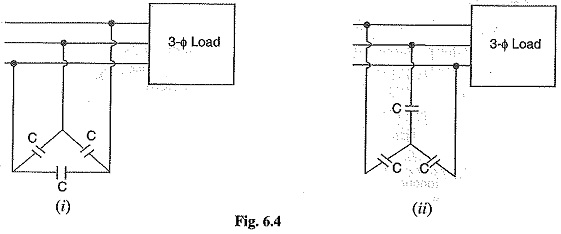• They have low losses.
• They require little maintenance as there are no rotating parts.
• They can be easily installed as they are light and require no foundation.
• They can work under ordinary atmospheric conditions.

• They have short service life ranging from 8 to 10 years.
• They are easily damaged if the voltage exceeds the rated value.
• Once the capacitors are damaged, their repair is uneconomical.

2.Synchronous condenser: A synchronous motor takes a leading current when over-excited and, therefore, behaves as a capacitor. An over-excited synchronous motor running on no load is known as synchronous condenser. When such a machine is connected in parallel with the supply, it takes a leading current which partly neutralizes the lagging reactive component of the load. Thus the power factor is improved.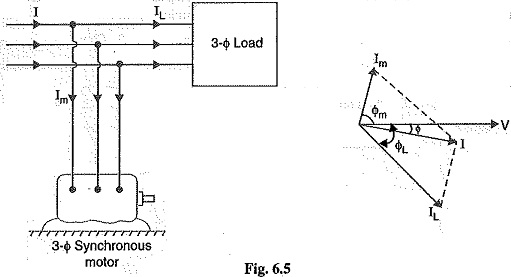Fig 6.5 shows the power factor improvement by synchronous condenser method. The 3Φ load takes current IL at low lagging power factor cos IL. The synchronous condenser takes a current Im which leads the voltage by an angle Φm. The resultant current I is the phasor sum of Im and IL and lags behind the voltage by an angle Φ. It is clear that Φ is less than ΦL, so that cos Φ is greater than cos ΦL. Thus the power factor is increased from cos ΦL to cos Φ. Synchronous condensers are generally used at major bulk supply substations for power factor improvement.

• By varying the field excitation, the magnitude of current drawn by the motor can be changed by any amount. This helps in achieving stepless control of power factor.
• The motor windings have high thermal stability to short circuit currents.
• The faults can be removed easily.

• There are considerable losses in the motor.
• The maintenance cost is high.
• It produces noise.
• Except in sizes above 500 kVA, the cost is greater than that of static capacitors of the same rating
• As a synchronous motor has no self-starting torque, therefore, an auxiliary equipment has to be provided for this purpose.

Note. The reactive power taken by a synchronous motor depends upon two factors, the d.c. field excitation and the mechanical load delivered by the motor. Maximum leading power is taken by a synchronous motor with maximum excitation and zero load.

3.Phase advancers: Phase advancers are used to improve the power factor of induction motors. The low power factor of an induction motor is due to the fact that its stator winding draws exciting current which lags be­hind the supply voltage by 90°. If the exciting ampere turns can be provided from some other a.c. source, then the stator winding will be relieved of exciting current and the power factor of the motor can be improved. This job is accomplished by the phase advancer which is simply an a.c. exciter. The phase advancer is mounted on the same shaft as the main motor and is connected in the rotor circuit of the motor. It provides exciting ampere turns to the rotor circuit at slip frequency. By providing more ampere turns than required, the induction motor can be made to operate on leading power factor like an over-excited synchronous motor.

Phase advancers have two principal advantages. Firstly, as the exciting ampere turns are sup­plied at slip frequency, therefore, lagging kVAR drawn by the motor are considerably reduced. Sec­ondly, phase advancer can be conveniently used where the use of synchronous motors is unadmissible. However, the major disadvantage of phase advancers is that they are not economical for motors below 200 H.P.

Updated: May 21, 2018 — 9:15 pm